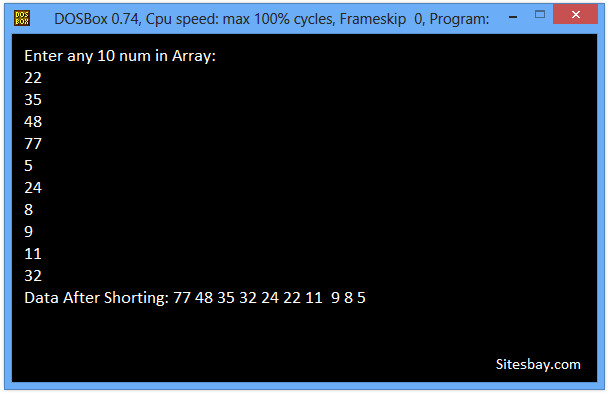# Sort an Array Elements in Descending Order in C

## Sort an Array Elements in Descending Order Program in C

Sort array elements in descending order means arrange elements of array in descending Order. If we want to sort an array, we have a wide variety of algorithms we can use to do the job. Three of the simplest algorithms are Selection Sort, Insertion Sort and Bubble Sort in C. None of these is especially efficient, but they are relatively easy to understand and to use.

## Output

```Elements Are: 5 3 77 23 4 90
After SOrt Elements Are: 3 4 5 23 77 90
```

A Sorting Algorithm is used to rearrange a given array or list elements according to a comparison operator on the elements. The comparison operator is used to decide the new order of element in the respective data structure.

### Different Ways to Sort Array Elements

There are literally hundreds of different ways to sort arrays. Below are the top ways to sort an array element in ascending or descending order.

• Bucket sort
• Bubble sort
• Insertion sort
• Selection sort
• Heapsort
• Mergesort

For sort array elements in decending order we print all elements of array from last index to first. For example arr, arr, arr,....arr

## Sort an Array Elements in Decending Order Program in C

```#include<stdio.h>
#include<conio.h>

void main()
{
int i,a,temp,j;
clrscr();
printf("Enter any 10 num in array : \n");
for(i=0;i<=10;i++)
{
scanf("%d",&a[i]);
}
printf("\n\nData before sorting  = ");
for(j=0;j<10;j++)
{
printf("  %d",a[j]);
}
for(i=0;i<=10;i++)
{
for(j=0;j<=10-i;j++)
{
if(a[j]>a[j+1])
{
temp=a[j];
a[j]=a[j+1];
a[j+1]=temp;
}
}
}
printf("\n\n\nData after sorting   : ");
for(j=9;j>=0 ;j--)
{
printf("  %d", a[j]);
}
getch();
}
```

## Output

```Enter any 10 num in Array:
22
35
48
77
5
24
8
9
11
32
Data After Shorting: 77 48 35 32 24 22 11  9 8 5
```WIFI CCTV Camera